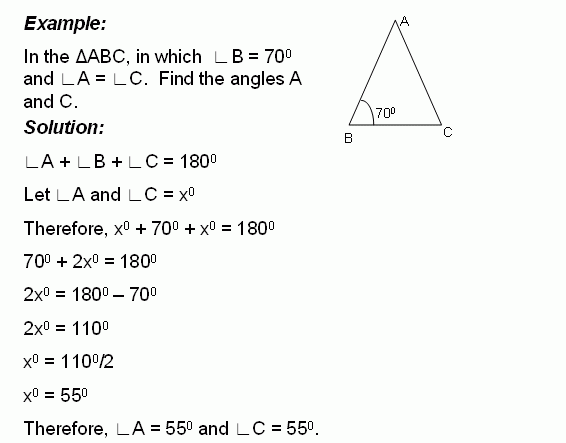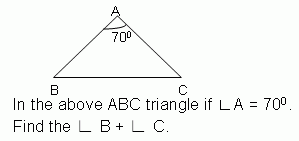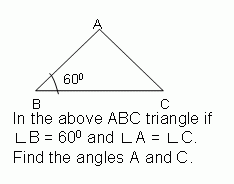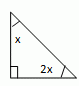Email us to get an instant 20% discount on highly effective K-12 Math & English kwizNET Programs!

#### Online Quiz (WorksheetABCD)

Questions Per Quiz = 2 4 6 8 10

### Middle/High School Algebra, Geometry, and Statistics (AGS)6.14 Find the Angles of TriangleDirections: Solve the following problems. Also write at least 10 examples of your own.
 Q 1:90 degrees100 degrees95 degrees Q 2:110 degrees105 degrees100 degrees Q 3:55 degrees60 degrees45 degrees Q 4: Find the angles of the triangle and classify based on the angles.45, 45, 9030, 60, 9020, 60, 100 Q 5: Find the angles of the triangle and classify based on the angles.32, 96, right angle triangle26, 64, right angle triangle30, 60, right angle triangle Q 6: Find the angles of the triangle.60, 60, 60 triangle30, 60, 90 triangle45, 45, 90 triangle Question 7: This question is available to subscribers only! Question 8: This question is available to subscribers only!